# Horizontal scaling

Horizontal scaling

There are various types of transformations of the graph of a function:

Operation Transformation of the Function f(x)
-f(x) reflection over x-axis
f(-x) reflection over y-axis
f(x) + k Translates up by k units
f(x) - k Translates down by k units
f(x + k) Translates left by k units
f(x - k) Translates right by k units
k f(x) Vertically scales the graph in y-axis (k > 1 stretch, 0 < k < 1 shrink vertical)
f(kx) Horizontally scales the graph in x-axis (k > 1 shrink, 0 < k < 1 stretch horizontal)

In this section, we will learn about horizontal scaling in detail and practice solving questions around it.

You can check out the interactive simulations to know more about the lesson and try your hand at solving a few interesting interactive questions at the end of the page.

## Lesson Plan

 1 What Do You Mean by Horizontal Scaling? 2 Important Notes on Horizontal Scaling 3 Solved Examples on Horizontal Scaling 4 Challenging Questions on Horizontal Scaling 5 Interactive Questions on Horizontal Scaling

## What Do You Mean by Horizontal Scaling?

Horizontal scaling means the stretching or shrinking the graph of the function along the x-axis.

Horizontal scaling can be done by multiplying the input with a constant.

Consider the following example:

Suppose, we have a function, $$y = f(x)$$

Horizontal scaling of the above function can be written as:$y = f(Cx)$

The graph stretches if the value of C < 1, and the graph will shink if the value of C > 1.

Coresponding points on the curves can be related from the table below:

The point on f(x) The point on y = f(Cx)
$$(x, y)$$

$$(Cx, y )$$

Let us understand this with an example:

Example

We have a function $$y = f(x)$$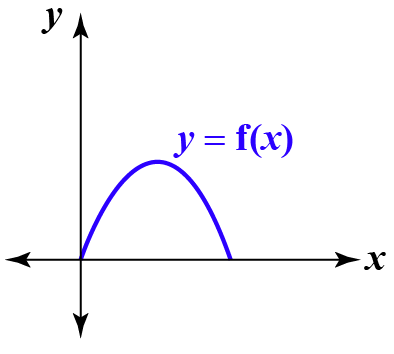We want to shrink the graph of the function, hence we have to multiply the input or x-coordinate by a constant greater than 1, let us multiply the function with 2, i.e., $y = f(2x)$

We get,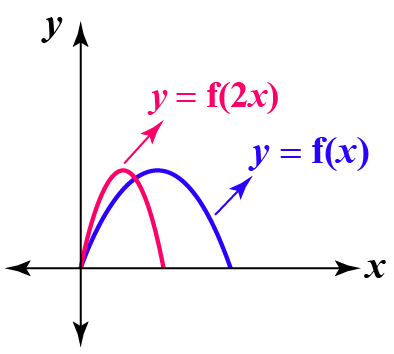We can see that the distance of the points on the curve gets closer to the y-axis.

## How Does One Horizontally Scale a Graph?

Steps

• Select the constant by which we want to scale the function.
• Write the new function as $$g(x) = fC(x)$$, where C is the constant.
• Trace the new function graph by replacing each value of x with Cx.
• X coordinate of each point in the graph is multiplied by $$\pm C$$, and the curve shrinks/stretches accordingly.

Let us understand this by an example:

Example

Suppose we have a basic quadratic equation $$f(x) = x^2$$ and the graphical representation of the graph is shown below.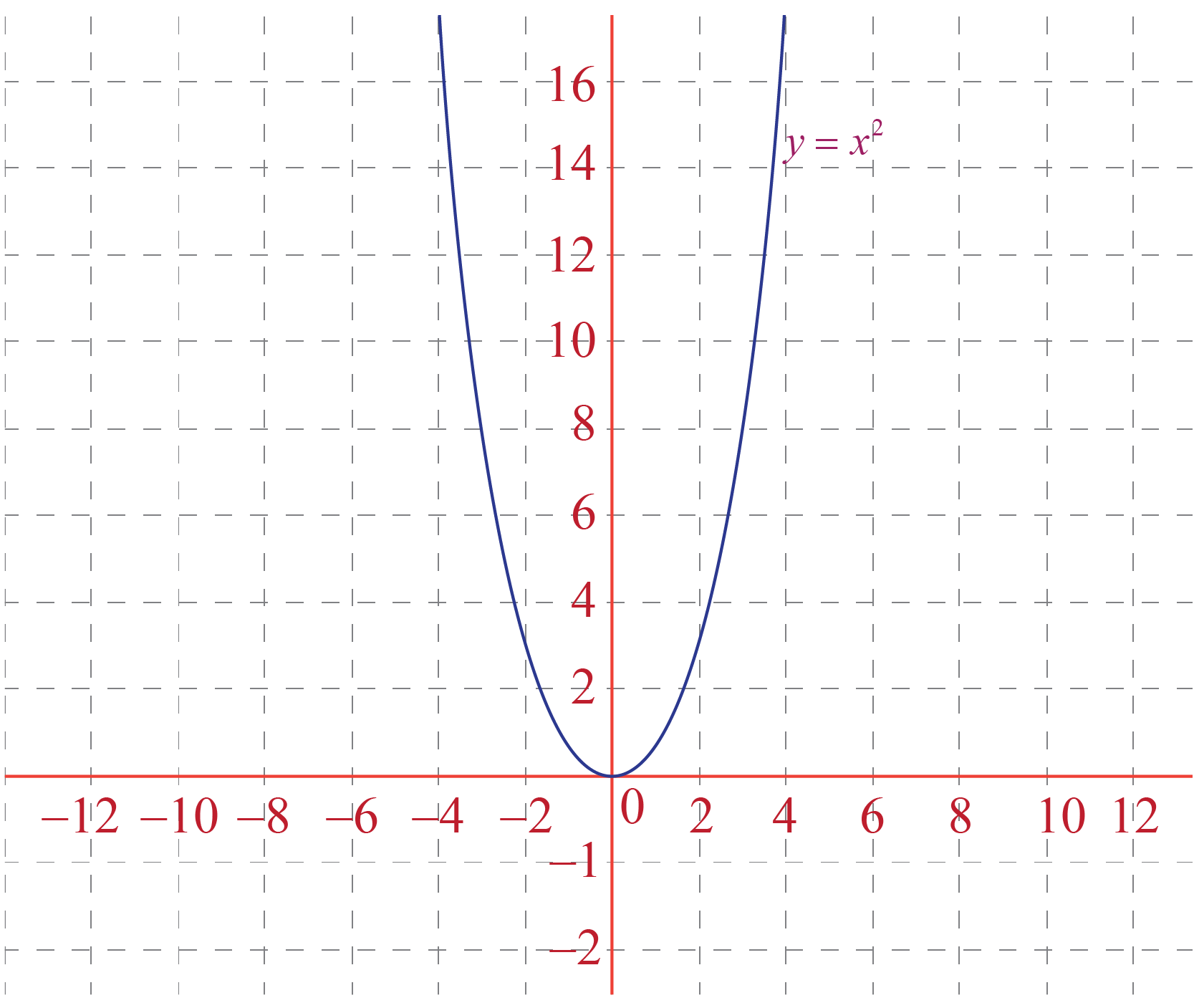We want to scale this function by a factor of $$\color{red}{+2}$$.

So our constant becomes $$\color{red}{+2}$$.

The equation of the new function will be:

$g(x) = \color{red}{2} f(x) = (\color{red}{2} x)^2$

Now, we have to replace the value of x-coordinate by $$\color{red}{2}x$$.

Hence we have to plot the graph of a function: $$f(x)=(2x)^2$$ and it is shrunk by a factor of $$\color{red}{+2}$$ units in the x-direction.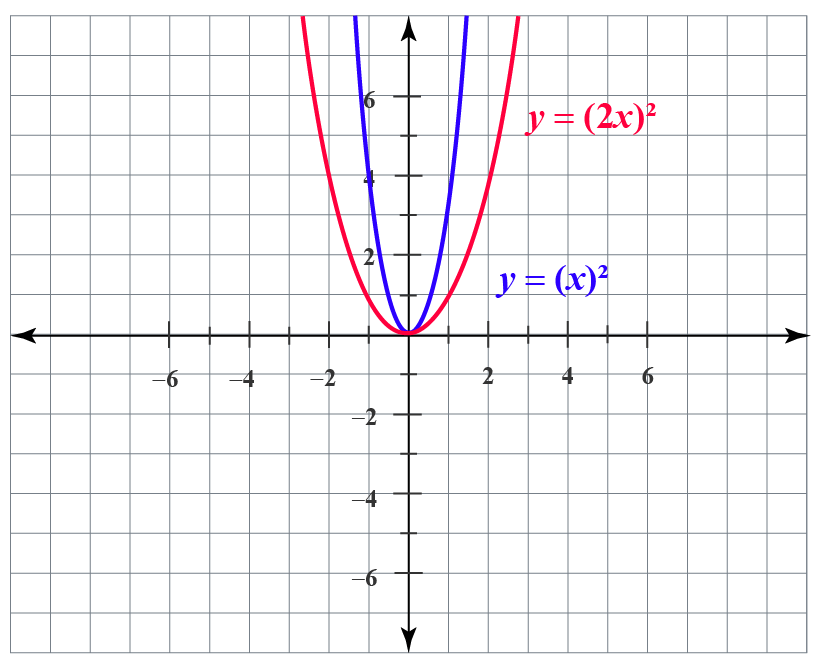Note: as we have scaled it with a factor of $$\color{red}{+2}$$ units, it has made the graph steeper.

## Horizontal Scaling in Graphs

Horizontal scaling of various graphs of the functions are shown below:

• Horizontal scaling of function f(x) = x+2 by a factor of 2 units is shown in the graph below: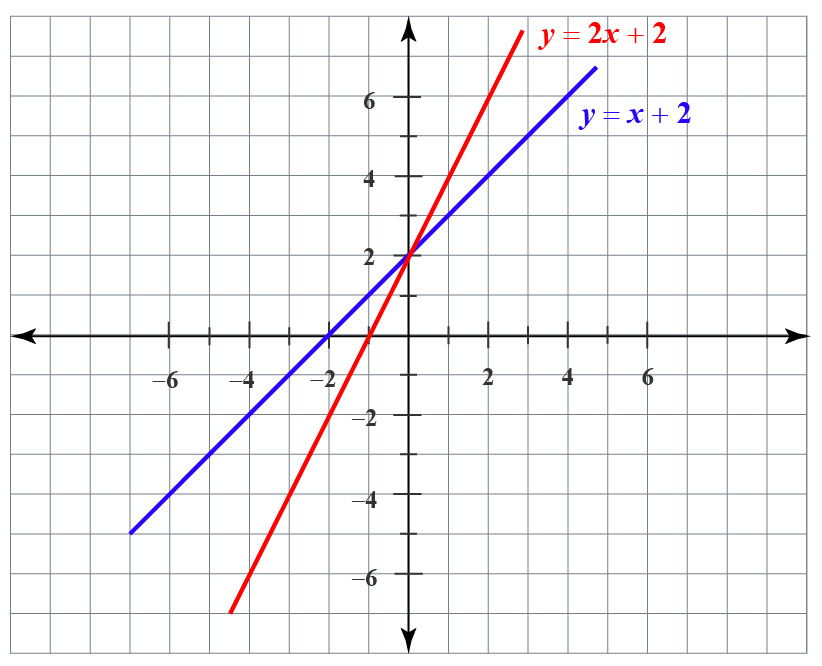• Horizontal scaling of function $$f(x) =(x^2 +3x+2)$$ by a factor of 4 units is shown in the graph below: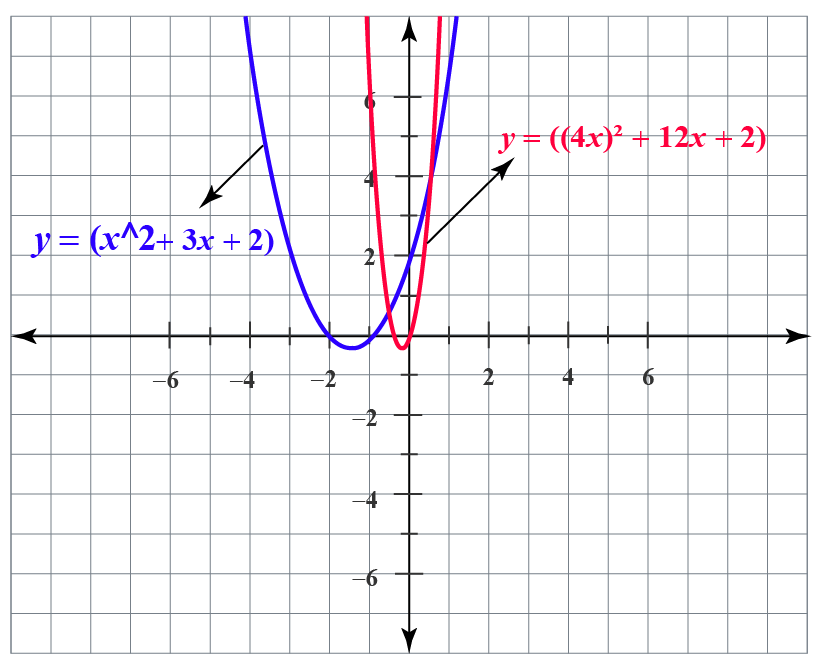• Horizontal scaling of function f(x) = sin x by a factor of -3, is shown in the graph below: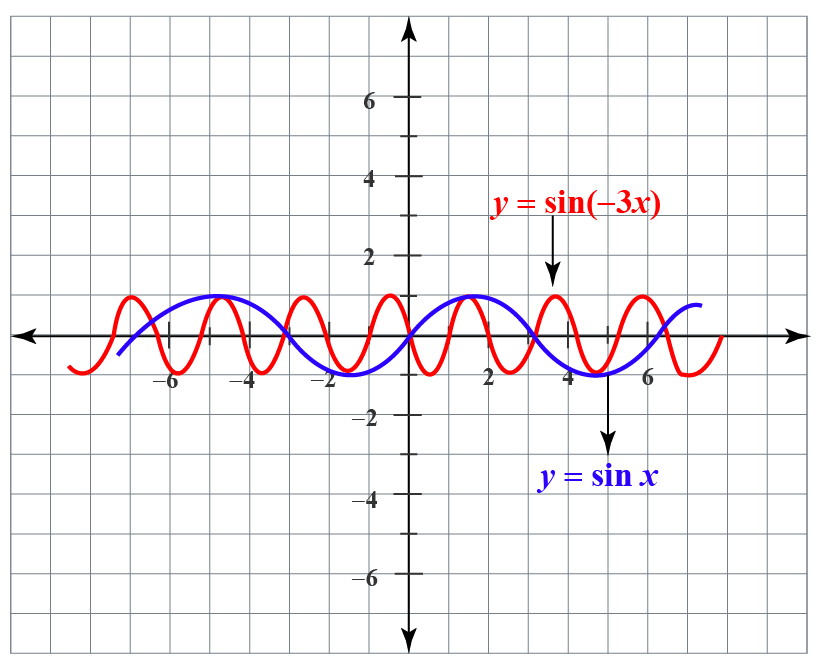1. Horizontal scaling refers to the shrinking or stretching of the curve along the x-axis by some specific units.
2. Horizontal scaling of function f(x) is given by g(x) =  $$\pm$$ f(Cx).
Functions
grade 10 | Questions Set 2
Functions